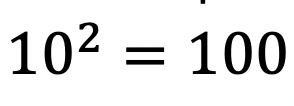## How to Solve Log Equations: Algebra 2/Trig.

Welcome to Mathsux! Today, we’re going to go over how to solve log equations, yay! But before we get into finding x, though, we need to go over what log equations are and why we use them in the first place…..just in case you were curious!

Also, if you have any questions about anything here, don’t hesitate to comment below or shoot me an email.  Happy calculating! 🙂

## What are Log Equations?

Logarithms are the inverses of exponential functions.  This means that when graphed, they are symmetrical along the line y=x.  Check it out below!

When on the same set of axis, notice how the functions are symmetrical over the line y=x:

We use logarithms to find the unknown values of exponents, such as the x value in the equation,.  This is a simple example, where we know the value of x is equal to 2,(). But what if it were to get more complicated?  That’s where logs come in!

## How to Solve Log Equations?

Logarithms follow a swooping pattern that allows us to write it in exponential form, let’s take a look at some Examples below:

But wait there’s more! Logs have a set of Rules that makes solving log equations a breeze!

We can use these rules to help us algebraically solve logarithmic equations, let’s look at an example that applies the Product Rule.

## Example:

Try the following practice questions on your own!

Practice Questions:

Solutions:

Still got questions?  No problem! Check out the video that goes over the same example outlined above.  And for more info. on logarithms check out this post that goes over a NYS Regent’s question here.  Subscribe below to get the latest FREE math videos, lessons, and practice questions from MathSux. Thanks for stopping by and happy calculating! 🙂

****Check out this Bonus Video on How to Change Log Bases****